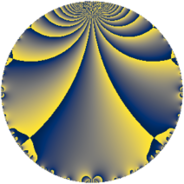Properties

 Label 14.3.dLevel $14$ Weight $3$ Character orbit 14.d Rep. character $\chi_{14}(3,\cdot)$ Character field $\Q(\zeta_{6})$ Dimension $4$ Newform subspaces $1$ Sturm bound $6$ Trace bound $0$

Related objects

Defining parameters

 Level: $$N$$ $$=$$ $$14 = 2 \cdot 7$$ Weight: $$k$$ $$=$$ $$3$$ Character orbit: $$[\chi]$$ $$=$$ 14.d (of order $$6$$ and degree $$2$$) Character conductor: $$\operatorname{cond}(\chi)$$ $$=$$ $$7$$ Character field: $$\Q(\zeta_{6})$$ Newform subspaces: $$1$$ Sturm bound: $$6$$ Trace bound: $$0$$

Dimensions

The following table gives the dimensions of various subspaces of $$M_{3}(14, [\chi])$$.

Total New Old
Modular forms 12 4 8
Cusp forms 4 4 0
Eisenstein series 8 0 8

Trace form

 $$4 q - 6 q^{3} - 4 q^{4} - 6 q^{5} + 8 q^{7} + O(q^{10})$$ $$4 q - 6 q^{3} - 4 q^{4} - 6 q^{5} + 8 q^{7} + 24 q^{10} + 18 q^{11} + 12 q^{12} - 36 q^{14} - 36 q^{15} - 8 q^{16} - 30 q^{17} - 24 q^{18} + 6 q^{19} + 54 q^{21} + 24 q^{22} + 30 q^{23} + 24 q^{24} + 4 q^{25} + 24 q^{26} - 20 q^{28} + 48 q^{29} - 12 q^{30} - 42 q^{31} - 90 q^{33} - 42 q^{35} - 62 q^{37} - 12 q^{38} + 12 q^{39} - 48 q^{40} + 72 q^{42} - 8 q^{43} + 36 q^{44} + 144 q^{45} + 36 q^{46} + 174 q^{47} - 20 q^{49} - 96 q^{50} + 54 q^{51} - 72 q^{52} - 78 q^{53} - 36 q^{54} + 48 q^{56} + 12 q^{57} + 24 q^{58} - 78 q^{59} + 36 q^{60} - 42 q^{61} - 216 q^{63} + 32 q^{64} - 84 q^{65} - 144 q^{66} - 58 q^{67} + 60 q^{68} + 84 q^{70} - 24 q^{71} - 48 q^{72} + 318 q^{73} + 96 q^{74} + 132 q^{75} + 126 q^{77} + 96 q^{78} + 110 q^{79} + 24 q^{80} + 18 q^{81} - 120 q^{82} + 12 q^{84} - 36 q^{85} + 24 q^{86} - 144 q^{87} - 24 q^{88} - 378 q^{89} + 24 q^{91} - 120 q^{92} - 138 q^{93} - 12 q^{94} - 30 q^{95} - 48 q^{96} - 120 q^{98} + 144 q^{99} + O(q^{100})$$

Decomposition of $$S_{3}^{\mathrm{new}}(14, [\chi])$$ into newform subspaces

Label Dim. $$A$$ Field CM Traces $q$-expansion
$a_{2}$ $a_{3}$ $a_{5}$ $a_{7}$
14.3.d.a $4$ $0.381$ $$\Q(\sqrt{2}, \sqrt{-3})$$ None $$0$$ $$-6$$ $$-6$$ $$8$$ $$q+\beta _{1}q^{2}+(-2-\beta _{1}-\beta _{2}+\beta _{3})q^{3}+\cdots$$